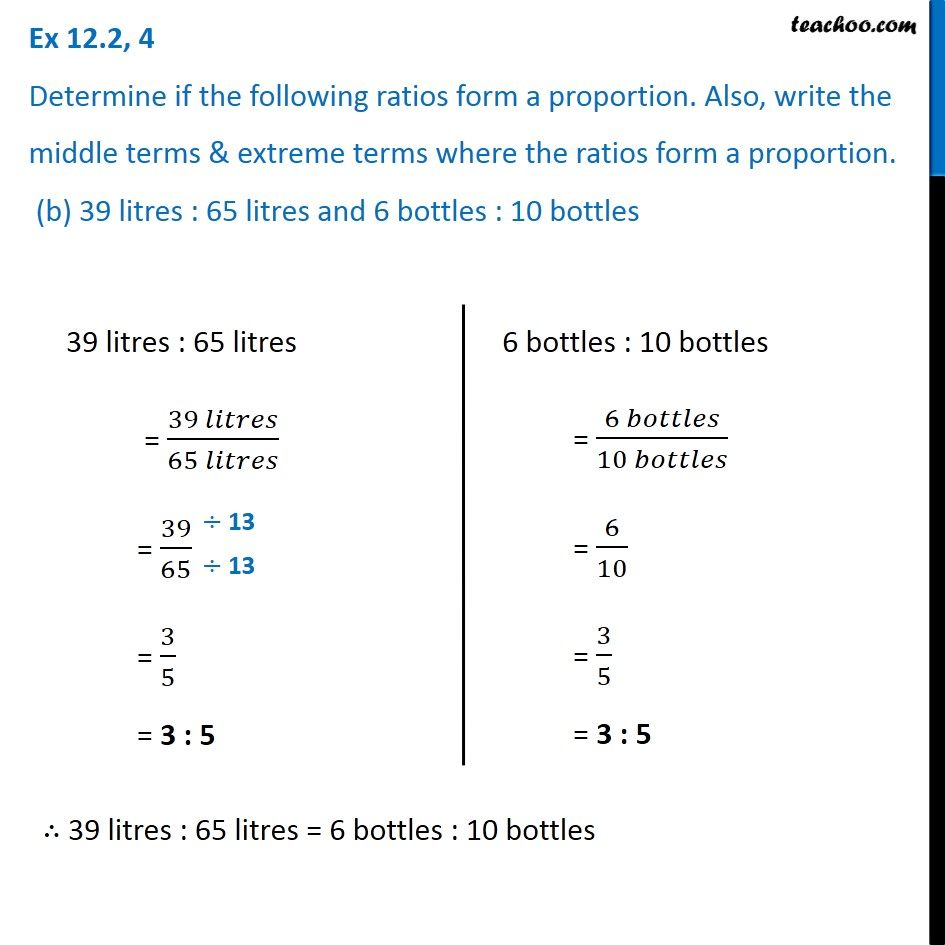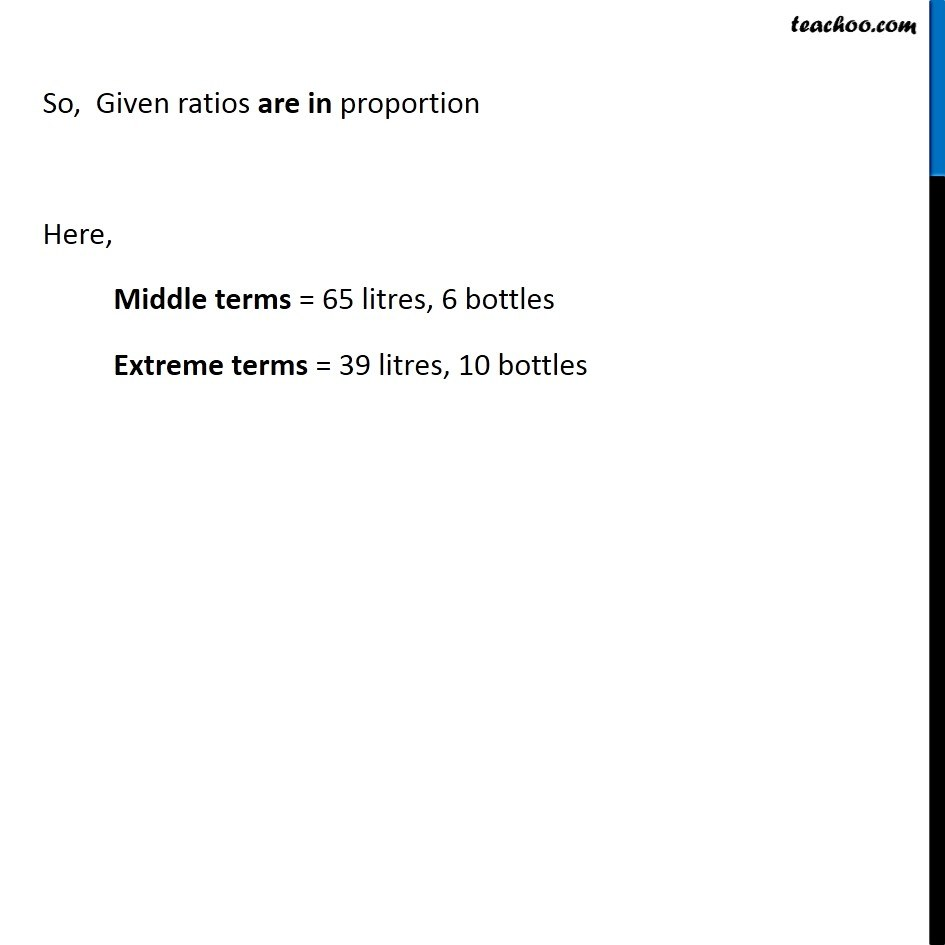Ex 12.2

Chapter 12 Class 6 Ratio and Proportion
Serial order wiseLearn in your speed, with individual attention - Teachoo Maths 1-on-1 Class

### Transcript

Ex 12.2, 4 Determine if the following ratios form a proportion. Also, write the middle terms & extreme terms where the ratios form a proportion. (b) 39 litres : 65 litres and 6 bottles : 10 bottles39 litres : 65 litres = (39 𝑙𝑖𝑡𝑟𝑒𝑠)/(65 𝑙𝑖𝑡𝑟𝑒𝑠) = 39/65 = 3/5 = 3 : 5 6 bottles : 10 bottles = (6 𝑏𝑜𝑡𝑡𝑙𝑒𝑠)/(10 𝑏𝑜𝑡𝑡𝑙𝑒𝑠) = 6/10 = 3/5 = 3 : 5 ∴ 39 litres : 65 litres = 6 bottles : 10 bottles So, Given ratios are in proportion Here, Middle terms = 65 litres, 6 bottles Extreme terms = 39 litres, 10 bottles